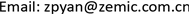JSTAJournal of Sensor Technology and Application2331-0235Scientific Research Publishing10.12677/JSTA.2019.72003JSTA-29576JSTA20190200000_19137757.pdf工程技术 应变计灵敏系数标定影响因素分析 Influence Factor Analysis for Strain Gage’s Gage Factor Calibration 志鹏1*中航电测仪器股份有限公司，陕西 汉中2603201907021927© Copyright 2014 by authors and Scientific Research Publishing Inc. 2014This work is licensed under the Creative Commons Attribution International License (CC BY). http://creativecommons.org/licenses/by/4.0/Copyright © 2019 by author(s) and Hans Publishers Inc.1. 引言

2. 应变计工作原理

R = ρ n l n s = ρ L S (1)

Δ R R = Δ L L − Δ S S + Δ ρ ρ (2)

Δ L L = ε

Δ S S = 2 Δ r r = − 2 μ Δ L L = − 2 μ ε

Δ R R = ( 1 + 2 μ ) ε + Δ ρ ρ (3)

K = Δ R / R ε = 1 + 2 μ + Δ ρ / ρ ε (4)

3. 应变计灵敏系数测量模型原理

3.1. 砝码加载四点式简支梁结构

M = F x − G 2 ( x − a ) = G 2 a (5)

ε = δ E = M W E = 6 F a E B h 2 = 3 G a E B h 2 (6)

(7)

ε = 12 h 3 L 1 2 − 4 a 3 × f (8)

3.2. 纯弯矩钢架梁结构

M = σ a (9)

ε ± = δ E = M W E = 6 σ a E B h 2 (10)

ε±为试验梁理论应变；σ为试验梁弯曲应力；E为试验梁弹性模量；W为试验梁抗弯截面系数，试验梁为矩形截面，W = Bh2/6。在实际状况下，试验梁还受一对非常小的轴向力，试验梁表面的凹、凸真实应变为：

ε = ( 1 + h 6 a ) × 6 σ a E B h 2 = ( 1 + h 6 a ) × ε ± (11)

ε = ( 1 − h 6 a ) × 6 σ a E B h 2 = ( 1 − h 6 a ) × ε ± (12)

ε + = h c 2 f + + f + − h − 1 3 μ h (13)

4. 不同测量方式对灵敏系数标定的影响因素分析和验证

4.1.常温灵敏系数测试

K = d R / R ε (15)

K = 2 ε s ε j (16)

4.1.1. 试验梁影响误差

ω s = 1 2 μ s 2 ε (17)

ω s = 1 2 × 0.3 × 5 2 × 0.001 = 0.375 % (18)

ω s = 1 2 × 0.3 × 1 2 × 0.001 = 0.15 % (19)

4.1.2. 不同标定装置测试常温灵敏系数误差验证

List of room temperature gage factor calibrated by different device

1f = 2.6028 ε = 1052 μm/m1128.32.145f = 2.4924 ε = 1000 μm/m10722.146
21141.92.1711072.52.145
31140.42.1681072.52.145
41132.52.15310712.142
51138.82.1651071.52.143
61137.22.16210722.144

4.2. 温度下灵敏系数测试4.2.1. 温度下试验梁载荷应变对灵敏系数测试的影响

ε = 6 F a E B h 2 = 3 G a E B h 2 (20)

ω j t = ( V G G ) 2 + ( V a a ) 2 + ( V B B ) 2 + 4 ( V h h ) 2 + ( V E E ) 2 (21)

List of linear expansion coefficient of 40CrMoNiA alloy steel test bea
20100200
10-6/℃11.311.511.9

List of elastic modulus of 40CrMoNiA alloy steel test bea
20100200
GPa209206203

ω j t = 0 + ( 420 × 11.9 × 10 − 6 × 180 420 ) 2 + 5 × ( 0.3 × 420 × 11.9 × 10 − 6 × 180 420 ) 2 + ( − 6 209 ) 2 = 0 + ( 11.9 × 10 - 6 × 180 ) 2 + 0.45 × ( 11.9 × 10 - 6 × 180 ) 2 + ( 0.0287 ) 2 = 2.88 %

ε = 3 G a E B h 2 = 3 × 150 × 5 209 × 10 4 × 3 × 0.6 2 = 997 × 10 − 6 (27)

ε 200 ˚ C = 3 G a E B h 2 = 3 × 150 × 5.025 203 × 10 4 × 2.9955 × 0.5991 2 = 1036 × 10 − 6 (28)

ω j t = ( 11.9 × 10 − 6 ) 2 + ( 0.3 × 11.9 × 10 − 6 ) 2 = 0.0012 %

f 凸 = − b + − b + 2 − ε + 2 c 2 2 ε + (29)

b + = − [ ε + h + ε + 1 3 μ h + ( 1 + h 6 a ) h ]

ε = ( 1 + h 6 a ) × h c 2 f + + f + − h − 1 3 μ h

ε = ( 1 + 9.6664 6 × 700 ) × 9.6664 150 2 2.3203 + 2.3203 − 9.6664 − 1 3 × 0.3 × 9.6664 = 1000.4 × 10 − 6

ε 200 ˚ C = ( 1 + 9.6395 6 × 700 ) × 9.6395 150.4177 2 2.3203 + 2.3203 − 9.6395 − 1 3 × 0.3 × 9.6395 = 991.7 × 10 − 6

4.2.2. 温度下不同试验梁结构标定灵敏系数随温度变化实际数据

Test data sheet of gage factor with temperature change by simply supported beam and steel frame beam structur

209971001000.4100
401002100.5997.499.7
801006100.9994.499.4
1201003100.6991.499.1
16099599.8985.498.5
20098498.7976.497.6
250969.197.2965.496.5

5. 结论